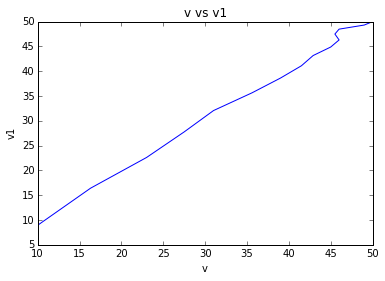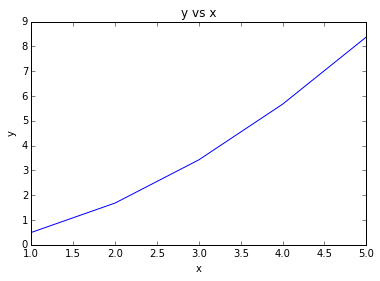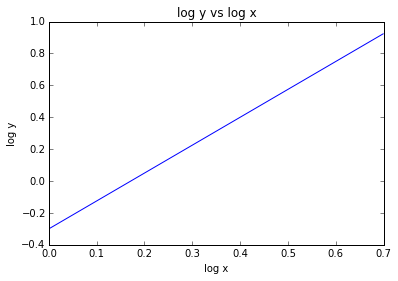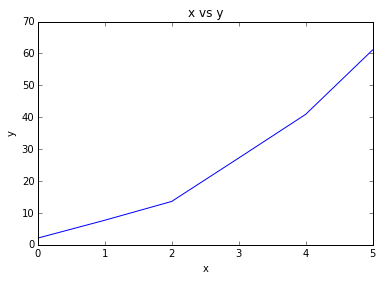# Chapter-17 : Least-squares Regression¶

## Ex:17.1 Pg: 458¶

In :
x = [1,2,3,4,5,6,7]#
y = [0.5,2.5,2,4,3.5,6,5.5]#
n = 7#
s = 0#
xsq = 0#
xsum = 0#
ysum = 0#

for i in range(0,7):
s = s + (x[i])*y[i]
xsq = xsq + x[i]**2
xsum = xsum + x[i]
ysum = ysum + y[i]

print "sum of product of x and y =",s
print "sum of square of x = ",xsq
print "sum of all the x = ",xsum
print "sum of all the y = ",ysum
a = xsum/n#
b = ysum/n#
a1 = (n*s - xsum*ysum)/(n*xsq -xsum**2)#
a0 = b - a*a1#
print "a1 = ",a1
print "a0 = ",a0
print "The equation of the line obtained is y = a0 + a1*x"

sum of product of x and y = 119.5
sum of square of x =  140
sum of all the x =  28
sum of all the y =  24.0
a1 =  0.839285714286
a0 =  0.0714285714286
The equation of the line obtained is y = a0 + a1*x


## Ex:17.2 Pg: 462¶

In :
x = [1,2,3,4,5,6,7]#
y = [0.5,2.5,2,4,3.5,6,5.5]#
n = 7#
s = 0#
ssum = 0#
xsq = 0#
xsum = 0#
ysum = 0#
msum = 0#
for i in range(0,7):
s = s + x[i]*y[i]
xsq = xsq + x[i]**2
xsum = xsum + x[i]
ysum = ysum + y[i]

a = xsum/n#
b = ysum/n#
a1 = (n*s - xsum*ysum)/(n*xsq -xsum**2)#
a0 = b - a*a1#
m=[];si=[]
for i in range(0,7):
m.append(y[i] - ysum/7**2)
msum = msum +m[i]#
si.append(y[i] - a0 - a1*x[i]**2)
ssum = ssum + si[i]

print "sum of all y =",ysum
print "\n(yi - yavg)**2 = ",m
print "\ntotal (yi - yavg)**2 = ",msum
print "\n(yi - a0 - a1*x)**2 = ",si
print "\ntotal (yi - a0 - a1*x)**2 = ",ssum
sy = (msum / (n-1))**(0.5)#
#sxy = (ssum/(n-2))**(0.5)#
print "\nsy = ",sy
#print "sxy = ",sxy
r2 = (msum - ssum)/(msum)#
r = r2**0.5#
print "r = ",r
print "The result indicate that 86.8 percent of the original uncertainty has been explained by linear model"

sum of all y = 24.0

(yi - yavg)**2 =  [0.010204081632653073, 2.010204081632653, 1.510204081632653, 3.510204081632653, 3.010204081632653, 5.510204081632653, 5.010204081632653]

total (yi - yavg)**2 =  20.5714285714

(yi - a0 - a1*x)**2 =  [-0.4107142857142855, -0.9285714285714284, -5.625, -9.5, -17.55357142857143, -24.285714285714285, -35.69642857142857]

total (yi - a0 - a1*x)**2 =  -94.0

sy =  1.85164019955
r =  2.35996704308
The result indicate that 86.8 percent of the original uncertainty has been explained by linear model


## Ex:17.3 Pg: 463¶

In :
%matplotlib inline
from matplotlib.pyplot import plot, title,xlabel,ylabel,show
from math import exp
s = [1,2,3,4,5,6,7,8,9,10,11,12,13,14,15]#
v = [10,16.3,23,27.5,31,35.6,39,41.5,42.9,45,46,45.5,46,49,50]#
g = 9.8#m/s**2
m = 68.1##kg
c = 12.5#kg/s
v1=[]
v2=[]
for i in range(0,15):
v1.append(g*m*(1 - exp(-c*s[(i)]/m))/c)
v2.append(g*m*s[(i)]/(c*(3.75+s[(i)])))

print "time = ",s
print "\nmeasured v =",v
print "\nusing equation(1.10) v1 = ",v1
print "\nusing equation((17.3)) v2 = ",v2
plot(v,v1)#
title('v vs v1')
xlabel('v')
ylabel('v1')#
show()

time =  [1, 2, 3, 4, 5, 6, 7, 8, 9, 10, 11, 12, 13, 14, 15]

measured v = [10, 16.3, 23, 27.5, 31, 35.6, 39, 41.5, 42.9, 45, 46, 45.5, 46, 49, 50]

using equation(1.10) v1 =  [8.953182207901257, 16.404980802870615, 22.607166909502304, 27.769291463870154, 32.06576523242002, 35.641751563129084, 38.61807096510561, 41.09528322582064, 43.15708498693595, 44.87313757134839, 46.30142060418256, 47.490190948641704, 48.479613142547706, 49.30311642251821, 49.988524185047744]

using equation((17.3)) v2 =  [11.240084210526316, 18.57057391304348, 23.729066666666665, 27.556335483870967, 30.5088, 32.855630769230764, 34.765841860465116, 36.35091063829787, 37.68734117647059, 38.82938181818182, 39.81656949152543, 40.678399999999996, 41.43732537313433, 42.11073802816901, 42.712320000000005]## Ex:17.4 Pg: 468¶

In :
from math import log10
%matplotlib inline
from matplotlib.pyplot import plot, title,xlabel,ylabel,show

#y = a*x**b
a1 = -0.3000#
a = 10**(a1)#
b = 1.75#
print "a=",a
x=[];y=[];m=[];n=[];
for i in range(0,5):
x.append(i+1)
y.append(a*x[(i)]**b)
m.append(log10(x[(i)]))
n.append(log10(y[(i)]))

print "x=",x
print "y=",y
print "m=",m
print "n=",n
plot(x,y)
title('y vs x')
xlabel('x')
ylabel('y')
show()
plot(m,n)
title('log y vs log x')
xlabel('log x')
ylabel('log y')
show()

a= 0.501187233627
x= [1, 2, 3, 4, 5]
y= [0.5011872336272722, 1.6857861925123965, 3.4273795077270295, 5.670286264671531, 8.379102586654781]
m= [0.0, 0.3010299956639812, 0.47712125471966244, 0.6020599913279624, 0.6989700043360189]
n= [-0.30000000000000004, 0.226802492411967, 0.5349621957594092, 0.7536049848239341, 0.9231975075880328]## Ex:17.5 Pg: 471¶

In :
%matplotlib inline
from matplotlib.pyplot import plot, title,xlabel,ylabel,show
from numpy import mat
x = [0,1,2,3,4,5]#
y = [2.1,7.7,13.6,27.2,40.9,61.1]#
sumy = 0#
sumx = 0#
m = 2#
n = 6#
xsqsum = 0#
xcsum = 0#
x4sum = 0#
xysum = 0#
x2ysum = 0#
rsum = 0#
usum = 0#
r=[];s=0
for i in range(0,6):
s = s + x[i]*y[i]
sumy = sumy+y[(i)]
sumx = sumx+x[(i)]
r.append((y[(i)] - s/n)**2)
xsqsum = xsqsum + x[(i)]**2
xcsum = xcsum +x[(i)]**3
x4sum = x4sum + x[(i)]**4
xysum = xysum + x[(i)]*y[(i)]
x2ysum = x2ysum + y[(i)]*x[(i)]**2
rsum = r[(i)] + rsum

print "sum y =",sumy
print "sum x =",sumx
xavg = sumx/n#
yavg = sumy/n#
print "xavg = ",xavg
print "yavg = ",yavg
print "sum x**2 =",xsqsum
print "sum x**3 =",xcsum
print "sum x**4 =",x4sum
print "sum x*y =",xysum
print "sum x**2 * y =",x2ysum
J = mat([[n,sumx,xsqsum],[sumx,xsqsum,xcsum],[xsqsum,xcsum,x4sum]])
I = mat([[sumy],[xysum],[x2ysum]])
X = (J**-1)* I
a0 = X[0,0]
a1 = X[1,0]
a2 = X[2,0]
u=[]
for i in range(0,6):
u.append((y[(i) ]- a0 - a1*x[(i)] - a2*x[(i)]**2)**2)
usum = usum + u[i]

print "(yi - yavg)**2 = ",r
print "(yi - a0 - a1*x - a2*x**2)**2 = ",u
plot(x,y)#
title('x vs y')
xlabel('x')
ylabel('y')
syx = (usum/(n-3))**0.5#
print "The standard error of the estimate based on regression polynomial =",syx
R2 = (rsum - usum)/(rsum)#
print "Percentage of original uncertainty that has been explained by the model = ",R2*100,'%'

sum y = 152.6
sum x = 15
xavg =  2
yavg =  25.4333333333
sum x**2 = 55
sum x**3 = 225
sum x**4 = 979
sum x*y = 585.6
sum x**2 * y = 2488.8
(yi - yavg)**2 =  [4.41, 41.173611111111114, 60.58027777777777, 60.580277777777745, 33.446944444444505, 1332.2500000000005]
(yi - a0 - a1*x - a2*x**2)**2 =  [0.14331632653056853, 1.0028591836732743, 1.0816000000005044, 0.80486530612177265, 0.61959387755180939, 0.094336734693502053]
The standard error of the estimate based on regression polynomial = 1.11752277062
Percentage of original uncertainty that has been explained by the model =  99.7555161238 %## Ex:17.6 Pg: 475¶

In :
from numpy import mat
x1 = [0,2,2.5,1,4,7]
x2 = [0,1,2,3,6,2]
x1sum = 0#
x2sum = 0#
ysum = 0#
x12sum = 0#
x22sum = 0#
x1ysum = 0#
x2ysum = 0#
x1x2sum = 0#
n = 6#
x12=[];x22=[];x1x2=[];x1y=[];x2y=[]
for i in range(0,6):
y.append(5 + 4*x1[(i)] - 3*x2[(i)])
x12.append(x1[(i)]**2)
x22.append(x2[(i)]**2)
x1x2.append(x1[(i)] * x2[(i)])
x1y.append(x1[(i)] * y[(i)])
x2y.append(x2[(i)] * y[(i)])
x1sum = x1sum + x1[(i)]
x2sum = x2sum + x2[(i)]
ysum = ysum + y[(i)]
x1ysum = x1ysum + x1y[(i)]#
x2ysum = x2ysum + x2y[(i)]#
x1x2sum = x1x2sum + x1x2[(i)]#
x12sum = x12sum + x12[(i)]#
x22sum = x22sum + x22[(i)]#

X = mat([[n,x1sum,x2sum],[x1sum,x12sum,x1x2sum],[x2sum,x1x2sum,x22sum]])
Y = mat([[ysum],[x1ysum],[x2ysum]])
Z = (X**-1)*Y
a0 = Z[0,0]
a1 = Z[1,0]
a2 = Z[2,0]
print "a0=",a0
print "a1=",a1
print "a2=",a2
print "Thus, y = a0 + a1*x1 + a2*x2"

a0= -0.850183257587
a1= 7.17727605923
a2= 2.80543175487
Thus, y = a0 + a1*x1 + a2*x2


## Ex:17.7 Pg: 479¶

In :
from numpy import mat,exp,transpose,shape,array
#y = -0.859 + 1.032*x
Z = mat([[1,10],[1,16.3],[1,23],[1,27.5],[1,31],[1,35.6],[1,39],[1,41.5],[1,42.9],[1,45],[1,46],[1,45.5],[1,46],[1,49],[1,50]])
Y=[]
for i in range(0,15):
Y.append(9.8*68.1*(1-exp(-12.5*(i+1)/68.1))/12.5)
Y=array(Y)
Y=Y.reshape(15,1)
M = transpose(Z)
R = M*Z#
S = M*Y#
P = (R**-1)#
X = (R**-1)*S#
a0 = X[0,0]
a1 = X[1,0]
print "a0=",a0
print "a1=",a1
sxy = 0.863403#
sa0 = ((P[0,0]) * sxy**2)**0.5
sa1 = (P[1,1] * sxy**2)**0.5
print "standard error of co efficient a0 = ",sa0
print "standard error of co efficient a1 = ",sa1
TINV = 2.160368#
a0 = [a0 - TINV*(sa0),a0 + TINV*(sa0)]#
a1 = [a1 - TINV*(sa1),a1 + TINV*(sa1)]#
print "interval of a0 = ",a0
print "interval of a1 = ",a1

a0= -0.858655686175
a1= 1.03160389481
standard error of co efficient a0 =  0.716371758333
standard error of co efficient a1 =  0.0186248847544
interval of a0 =  [-2.4062823089821084, 0.68897093663270859]
interval of a1 =  [0.99136728978321009, 1.0718404998372961]


## Ex:17.8 Pg: 481¶

In :
from math import exp
from numpy import array,transpose,mat
x = [0.25,0.75,1.25,1.75,2.25]#
y = [0.28,0.57,0.68,0.74,0.79]#
a0 = 1#
a1 = 1#
sr = 0.0248#
pda0=[];pda1=[]
for i in range(0,5):
pda0.append(1 - exp(-a1 * x[(i)]))
pda1.append(a0 * x[(i)]*exp(-a1*x[(i)]))

Z0 = mat([[pda0,pda1],[pda0,pda1],[pda0,pda1],[pda0,pda1],[pda0,pda1]])
print "Z0 = ",Z0
R = transpose(Z0)*Z0
S = (R**-1)
y1=[];D=[]
for i in range(0,5):
y1.append(a0 * (1-exp(-a1*x[(i)])))
D.append(y[(i)] - y1[(i)])
D=array(D)
D=D.reshape(5,1)
print "D = ",D
M = transpose(Z0)*D
X = S *M#
print "X = ",X
a0 = a0 + X[0,0]
a1 = a1 + X[1,0]
print "The value of a0 after 1st iteration = ",a0
print "The value of a1 after 1st iteration = ",a1

Z0 =  [[ 0.22119922  0.1947002 ]
[ 0.52763345  0.35427491]
[ 0.7134952   0.358131  ]
[ 0.82622606  0.3041044 ]
[ 0.89460078  0.23714826]]
D =  [[ 0.05880078]
[ 0.04236655]
[-0.0334952 ]
[-0.08622606]
[-0.10460078]]
X =  [[-0.27147736]
[ 0.50193087]]
The value of a0 after 1st iteration =  0.728522640015
The value of a1 after 1st iteration =  1.5019308677